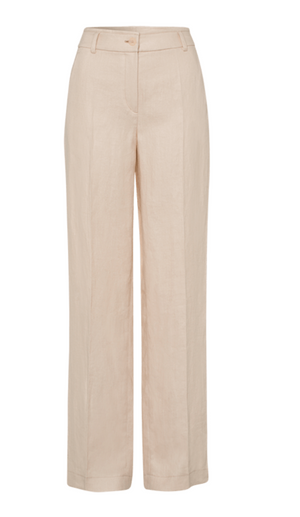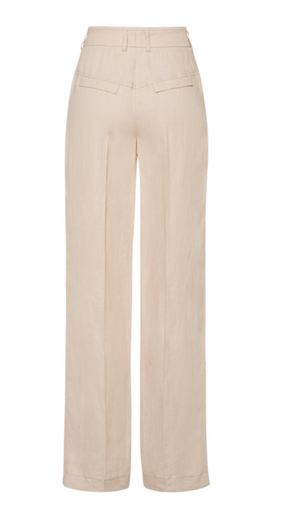Riani

# Linen Culottes

Regular price \$398 now \$278.60 Unit price  per

Shipping calculated at checkout.
• 100% linen
• Machine wash cold. Hang to dry
• Dry clean
• High rise
• Fits true to size
• Looser fit in the legs
• Slanted pockets

Size Guide

• 34 = 4 = XS
• 36 = 6 = S
• 38 = 8 = M
• 40 = 10 = M
• 42 = 12 = L
• 44 = 14 = L
• 46 = 16 = XL